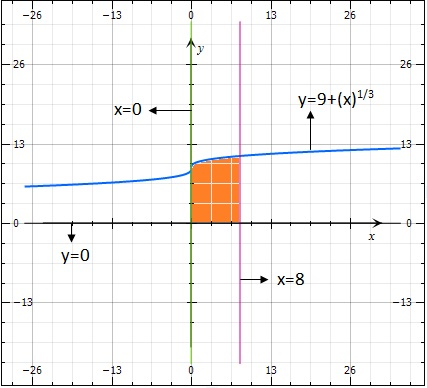# Find the area of the region bounded by the graphs of the equations y = 9 + \sqrtx, x=0,x=8,y=0

## Question:

Find the area of the region bounded by the graphs of the equations {eq}y = 9 + \sqrtx, x=0,x=8,y=0 {/eq}

## Area using integrals:

In the given problem, we will find area of the region bounded by the curves. Here the limits of the integral are already defined from {eq}x=0 {/eq} to {eq}x=8 {/eq}, so we do not require to find the points of intersection.

Given {eq}y = 9 + \sqrtx, x=0,x=8,y=0 {/eq}

Graph for the given curves:The function {eq}y=9 + \sqrtx {/eq} is forming the upper...

Become a Study.com member to unlock this answer! Create your account